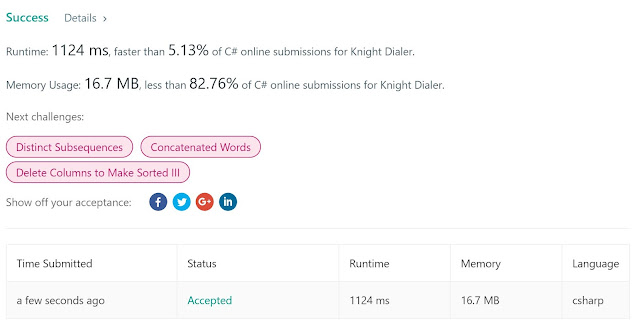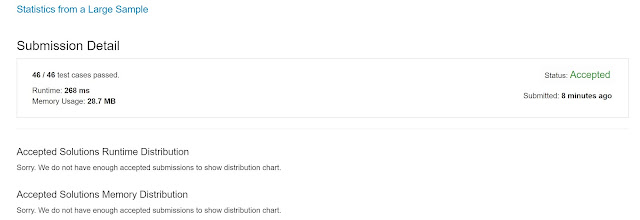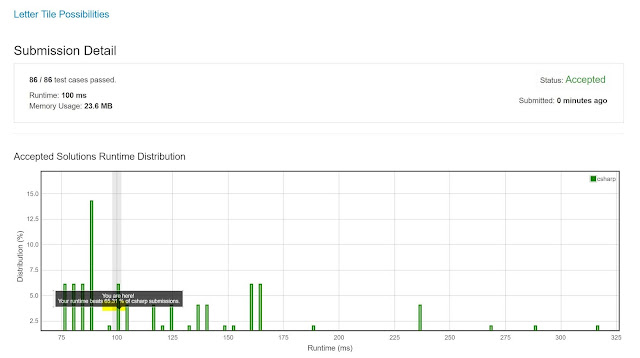## Posts

Showing posts from June, 2019

### Reverse Polish Notation - A Stack Application

Medium L.C. problem:  https://leetcode.com/problems/evaluate-reverse-polish-notation/ Evaluate the value of an arithmetic expression in  Reverse Polish Notation . Valid operators are  + ,  - ,  * ,  / . Each operand may be an integer or another expression. Note: Division between two integers should truncate toward zero. The given RPN expression is always valid. That means the expression would always evaluate to a result and there won't be any divide by zero operation. Example 1: Input: ["2", "1", "+", "3", "*"] Output: 9 Explanation: ((2 + 1) * 3) = 9 Example 2: Input: ["4", "13", "5", "/", "+"] Output: 6 Explanation: (4 + (13 / 5)) = 6 Example 3: Input: ["10", "6", "9", "3", "+", "-11", "*", "/", "*", "17", "+", "5", "+"] Outp

### Knight Dialer - A Classic Dynamic Programming ProblemThis was a very interesting problem, and a classic application of Dynamic Programming. Here is the problem: https://leetcode.com/problems/knight-dialer/ A chess knight can move as indicated in the chess diagram below: This time, we place our chess knight on any numbered key of a phone pad (indicated above), and the knight makes  N-1  hops.  Each hop must be from one key to another numbered key. Each time it lands on a key (including the initial placement of the knight), it presses the number of that key, pressing  N  digits total. How many distinct numbers can you dial in this manner? Since the answer may be large,  output the answer modulo  10^9 + 7 . Example 1: Input: 1 Output: 10 Example 2: Input: 2 Output: 20 Example 3: Input: 3 Output: 46 Note: 1 <= N <= 5000 The 10^9 + 7 to me is always the strongest indication that the solution should be done via DP. The also indication is that the N is very large (5000), hence if we try all the

### Statistics from a Large SampleWhat I like about this problem is that it allows one to review some basic statistic concepts that will be needed for the rest of a professional mathematician or computer scientist career. Here it is:  https://leetcode.com/problems/statistics-from-a-large-sample/ We sampled integers between  0  and  255 , and stored the results in an array  count :   count[k]  is the number of integers we sampled equal to  k . Return the minimum, maximum, mean, median, and mode of the sample respectively, as an array of  floating point numbers .  The mode is guaranteed to be unique. (Recall that the median of a sample is: The middle element, if the elements of the sample were sorted and the number of elements is odd; The average of the middle two elements, if the elements of the sample were sorted and the number of elements is even.) Getting the min and max is very easy, so we'll skip explanation. Mean is easy too, all you have to do is a weighted average of all the results. The mode if

### Permutation with no RepetitionThis is a classic problem with a well-established technique for solving it. Comes from Leetcode: https://leetcode.com/problems/letter-tile-possibilities/ You have a set of  tiles , where each tile has one letter  tiles[i]  printed on it.  Return the number of possible non-empty sequences of letters you can make. Example 1: Input: "AAB" Output: 8 Explanation: The possible sequences are "A", "B", "AA", "AB", "BA", "AAB", "ABA", "BAA". Example 2: Input: "AAABBC" Output: 188 Note: 1 <= tiles.length <= 7 tiles  consists of uppercase English letters. The solution (or better, one of the solutions) is as follows:  - Do a standard DFS using the "output position" to control the recursion  - Have a global hash table tracking the index  that has already been used  - Have a local  hash table tracking the character  that has already been used  - That